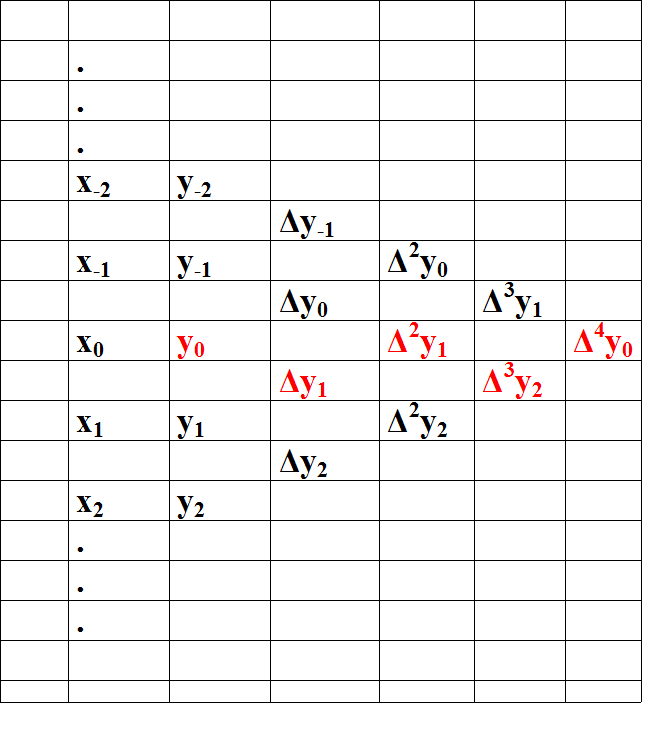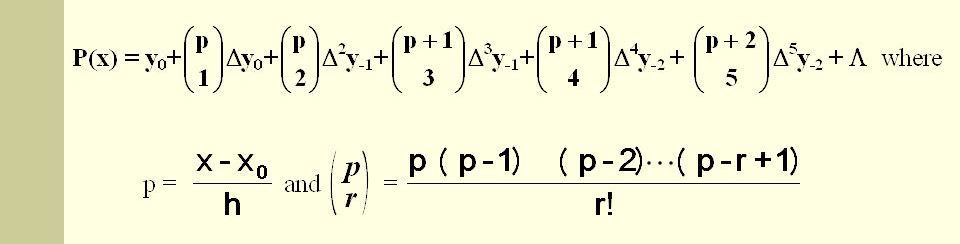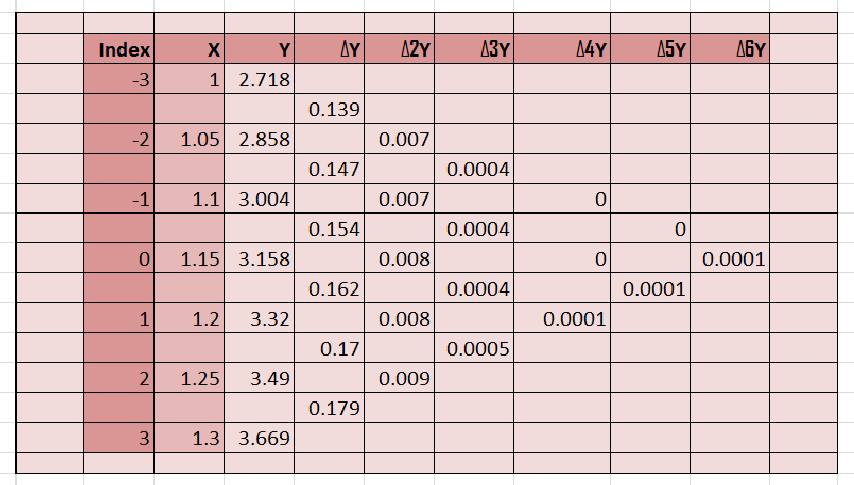# Gauss’s Forward Interpolation

Interpolation refers to the process of creating new data points given within the given set of data. The below code computes the desired data point within the given range of discrete data sets using the formula given by Gauss and this method known as Gauss’s Forward Method.

# Gauss’s Forward Method:

The gaussian interpolation comes under the Central Difference Interpolation Formulae. Suppose we are given the following value of y=f(x) for a set values of x:
X: x0 x1 x2 ………. xn
Y: y0 y1 y2 ………… yn
The differences y1 – y0, y2 – y1, y3 – y2, ……, yn – yn–1 when denoted by Δy0, Δy1, Δy2, ……, Δyn–1 are respectively, called the first forward differences. Thus the first forward differences are :

Δy0 = y1 – y0

and in the same way we can calculate higher order differences.And after the creating table we calculate the value on the basis of following formula:Now, Let’s take an example and solve it for better understanding.
Problem:
From the following table, find the value of e1.17 using Gauss’s Forward formula.

 x 1.00 1.05 1.10 1.15 1.20 1.25 1.30 ex 2.7183 2.8577 3.0042 3.1582 3.3201 3.4903 3.6693

Solution:
We have

yp = y0 + pΔy0 + (p(p-1)/2!).Δy20 + ((p+1)p(p-1)/3!).Δy30 + …

where p = (x1.17 – x1.15) / h
and h = x1 – x0 = 0.05
so, p = 0.04

Now, we need to calculate Δy0, Δy20, Δy30 … etc.Put the required values in the formula-
yx = 1.17 = 3.158 + (2/5)(0.162) + (2/5)(2/5 – 1)/2.(0.008) …
yx = 1.17 = 3.2246

Code : Python code for implementing Gauss’s Forward Formula

 `# Python3 code for Gauss's Forward Formula ` `# importing library ` `import` `numpy as np ` ` `  `# function for calculating coefficient of Y  ` `def` `p_cal(p, n):  ` ` `  `    ``temp ``=` `p;  ` `    ``for` `i ``in` `range``(``1``, n):  ` `         ``if``(i``%``2``=``=``1``): ` `             ``temp ``*` `(p ``-` `i) ` `         ``else``: ` `             ``temp ``*` `(p ``+` `i) ` `    ``return` `temp;  ` `# function for factorial ` `def` `fact(n):  ` `    ``f ``=` `1`  `    ``for` `i ``in` `range``(``2``, n ``+` `1``):  ` `        ``f ``*``=` `i ` `    ``return` `f  ` ` `  `# storing available data ` `n ``=` `7``;  ` `x ``=` `[ ``1``, ``1.05``, ``1.10``, ``1.15``, ``1.20``, ``1.25``, ``1.30` `];  ` ` `  `y ``=` `[[``0` `for` `i ``in` `range``(n)]  ` `        ``for` `j ``in` `range``(n)];  ` `y[``0``][``0``] ``=` `2.7183``;  ` `y[``1``][``0``] ``=` `2.8577``;  ` `y[``2``][``0``] ``=` `3.0042``; ` `y[``3``][``0``] ``=` `3.1582``;   ` `y[``4``][``0``] ``=` `3.3201``;  ` `y[``5``][``0``] ``=` `3.4903``;  ` `y[``6``][``0``] ``=` `3.6693``;  ` ` `  `# Genrating Gauss's triangle ` `for` `i ``in` `range``(``1``, n):  ` `    ``for` `j ``in` `range``(n ``-` `i):  ` `        ``y[j][i] ``=` `np.``round``((y[j ``+` `1``][i ``-` `1``] ``-` `y[j][i ``-` `1``]),``4``);  ` ` `  `# Printing the Triangle  ` `for` `i ``in` `range``(n):  ` `    ``print``(x[i], end ``=` `"\t"``);  ` `    ``for` `j ``in` `range``(n ``-` `i):  ` `        ``print``(y[i][j], end ``=` `"\t"``);  ` `    ``print``("");  ` ` `  `# Value of Y need to predict on  ` `value ``=` `1.17``;  ` ` `  `# implementing Formula ` `sum` `=` `y[``int``(n``/``2``)][``0``];  ` `p ``=` `(value ``-` `x[``int``(n``/``2``)]) ``/` `(x[``1``] ``-` `x[``0``]) ` ` `  `for` `i ``in` `range``(``1``,n):  ` `    ``# print(y[int((n-i)/2)][i]) ` `    ``sum` `=` `sum` `+` `(p_cal(p, i) ``*` `y[``int``((n``-``i)``/``2``)][i]) ``/` `fact(i) ` ` `  `print``(``"\nValue at"``, value,  ` `    ``"is"``, ``round``(``sum``, ``4``));  `

Output :

```1       2.7183  0.1394  0.0071  0.0004  0.0     0.0     0.0001
1.05    2.8577  0.1465  0.0075  0.0004  0.0     0.0001
1.1     3.0042  0.154   0.0079  0.0004  0.0001
1.15    3.1582  0.1619  0.0083  0.0005
1.2     3.3201  0.1702  0.0088
1.25    3.4903  0.179
1.3     3.6693

Value at 1.17 is 3.2246```

My Personal Notes arrow_drop_upCheck out this Author's contributed articles.

If you like GeeksforGeeks and would like to contribute, you can also write an article using contribute.geeksforgeeks.org or mail your article to contribute@geeksforgeeks.org. See your article appearing on the GeeksforGeeks main page and help other Geeks.

Please Improve this article if you find anything incorrect by clicking on the "Improve Article" button below.

Be the First to upvote.

Please write to us at contribute@geeksforgeeks.org to report any issue with the above content.